### LOG Function Examples – Excel, VBA, & Google Sheets

This Tutorial demonstrates how to use the Excel LOG Function in Excel to calculate the logarithm of a number to a specified base.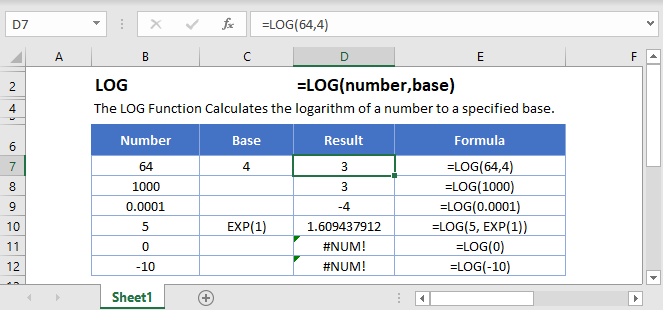## LOG Function Overview

The LOG Function Calculates the logarithm of a number to a specified base.

To use the LOG Excel Worksheet Function, select a cell and type: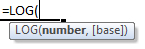(Notice how the formula inputs appear)

## LOG Function Syntax and Inputs:

number – A number.

base – OPTIONAL. The base of the logarithm. Blank as default.

AutoMacro - VBA Code Generator

## LOG Function

The LOG Function returns the logarithm of a number to the specified base.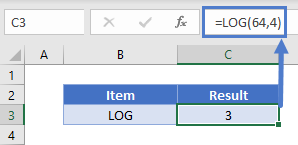## LOG Function – Base 10

The LOG Function will return the logarithm of a number to base 10 if the second argument is omitted.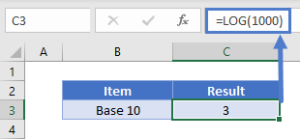Alternatively, the LOG10 Function can also be used. To learn more, click here.

## LOG Function – Base ‘e’

The LOG Function can return the logarithm of a number to base ‘e’.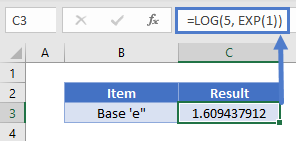Alternatively, the LN Function can also be used. To learn more, click here.

## LOG & POWER Function

The LOG Function is a reverse of the POWER Function. The LOG Function returns the power to which the given base was raised.

Let’s say we raise 7 to the power of 3.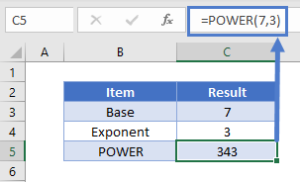The resulting number when entered in the LOG Function to the base 7 will return the power.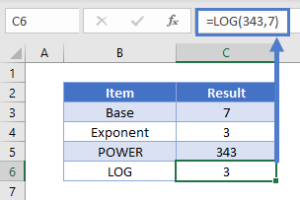To learn more about the POWER Function, click here.

## LOG Function – Negative Number/Zero

The LOG Function will return an error if the argument is zero or a negative number.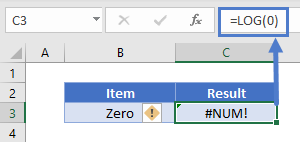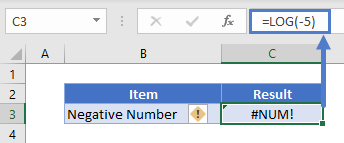## LOG in Google Sheets

The LOG Function works exactly the same in Google Sheets as in Excel: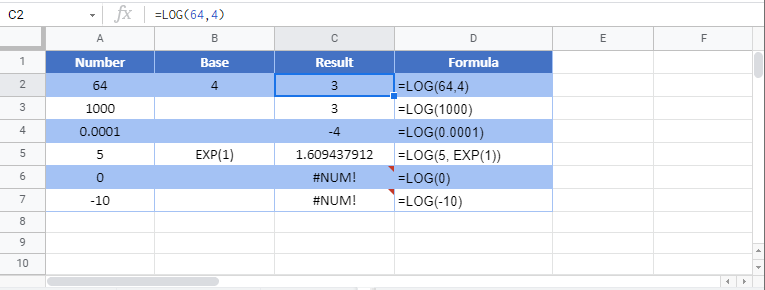## Additional Notes

Use the LOG Function to calculate the logarithm of a number to a specified base. Simply enter any number directly into the formula, or reference a cell containing a number.

## LOG Examples in VBA

You can also use the LOG function in VBA. Type:
`application.worksheetfunction.log(number,base)`
For the function arguments (number, etc.), you can either enter them directly into the function, or define variables to use instead.

Return to the List of all Functions in Excel

## Excel Practice Worksheet

Practice Excel functions and formulas with our 100% free practice worksheets!

• Automatically Graded Exercises
• Learn Excel, Inside Excel!